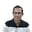Related Tags

golang
communitycreator

# How to communicate signals across goroutines with channelsJeyabalaji Subramanian

### What is a Channel?

As per the golang documentation, “Channels are a typed conduit through which you can send and receive values with the channel operator, <-.”

A channel allows one goroutine to signal another goroutine about a particular event.

Before going any further, let’s look at a quick example where channels are used to get the sum of a slice by concurrently distributing the work across two goroutines.

package main

import "fmt"

func sum(s []int, c chan int) {
sum := 0
for _, v := range s {
sum += v
}
c <- sum // send sum to c
}

func main() {
s := []int{7, 2, 8, -9, 4, 0}

c := make(chan int)
go sum(s[:len(s)/2], c)
go sum(s[len(s)/2:], c)
x, y := <-c, <-c // receive from c

fmt.Println(x, y, x+y)
}

### Synchronous channels

In the above example, with the c := make(chan int) command, we created and used an unbuffered or synchronous channel.

Below are the characteristics of an unbuffered channel:

If a channel is unbuffered, the sender blocks until the receiver has received the value. Receivers always block until there is data to receive.

So, in the above example, the x, y := <-c, <-c command blocks until there is data in the channel sent by the sum(s []int, c chan int) goroutine function.

What happens when a goroutine sends data to a channel, but there is no receiver?

Let’s look at the answer for this question with in the small code example below:

package main
import "fmt"
/* For exercises uncomment the imports below */
// import "strconv"
// import "encoding/json"
func main() {
fmt.Printf("Hello World")
c := make(chan int)

c <- 5
}

In the above code example, we get a deadlock error when data is sent to a channel with no receiver.

An unbuffered channel is a synchronous channel, i.e., a sender and a receiver must be simultaneously present.

RELATED TAGS

golang
communitycreator

CONTRIBUTORJeyabalaji Subramanian